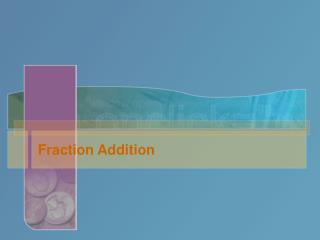DownloadDownload PresentationDownload Presentation- - - - - - - - - - - - - - - - - - - - - - - - - - - E N D - - - - - - - - - - - - - - - - - - - - - - - - - - -
##### Presentation Transcript

2. ¾ + ¾ • Count the pieces

3. Try some • 4/5 + 3/5

4. Compare

5. Try some

6. Apples and Oranges • The things you add have to be the same size

7. Common Denominator

8. Common Denominator • A number all original denominators divide into evenly • 12 is a common denominator because 3 and 4 both divide 12 evenly.

9. Least Common Denominator • To find the least common denominator • Write the times table list for each denominator • The smallest number that is in all rows is the least common denominator

10. Least Common Denominator • To find the least common denominator • Write the times table list for each denominator • The smallest number that is in all rows is the least common denominator

11. Try One • Find the common denominator for these fractions • A) 2/3 + 3/5 • B)¾ + 7/12 • C)2/5 + 3/8 + 7/10